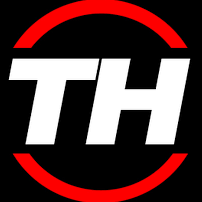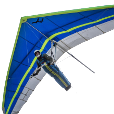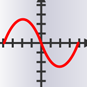# All Activity

1. Past hour
2.## LISP to change object colors on a drawing

OK. Try this (defun rh:getlocked ( doc / lst) (vlax-for lyr (vla-get-layers doc) (cond ( (= :vlax-true (vlax-get-property lyr 'lock)) (setq lst (cons (list lyr) lst)) (vlax-put-property lyr 'lock :vlax-false) ) );end_cond );end_for (if lst (setq lst (reverse lst)) (setq lst nil)) );end_defun (vl-load-com) (defun c:c151 (/ *error* clst c_doc llst ocnt ss cnt ent el typ obj) (defun *error* ( msg ) (mapcar '(lambda (x) (vlax-put-property x 'lock :vlax-true)) llst) (if (not (wcmatch (strcase msg) "*BREAK*,*CANCEL*,*EXIT*")) (princ (strcat "\nOops an Error : " msg " occurred."))) (princ) );_end_*error*_defun (setq clst (list 1 2 3 4 5 6 7 8 9 40 41 80 140) c_doc (vla-get-activedocument (vlax-get-acad-object)) llst (rh:getlocked c_doc) ocnt 0 );end_setq (vlax-for lyr (vla-get-layers c_doc) (cond ( (vl-position (vlax-get lyr 'color) clst) (vlax-put lyr 'color 151) (setq ocnt (1+ ocnt)))) (setq lylst (cons (list (vlax-get lyr 'name) (vlax-get lyr 'color)) lylst)) );end_for (princ (strcat "\n" (itoa ocnt) "Layer Colors Changed")) (setq ocnt 0 ss (ssget "_X" '((-4 . "<NOT") (62 . 256) (-4 . "NOT>"))) );end_setq (cond (ss (repeat (setq cnt (sslength ss)) (setq el (entget (setq ent (ssname ss (setq cnt (1- cnt))))) typ (cdr (assoc 0 el)) lyr (cdr (assoc 8 el)) );end_setq (cond ( (not (member typ (list "INSERT" "TABLE"))) (setq obj (vlax-ename->vla-object ent)) (cond ( (vl-position (vlax-get obj 'color) clst) (if (= (cadr (assoc lyr lylst)) 151) (vlax-put obj 'color 256) (vlax-put obj 'color 151)) (setq ocnt (1+ ocnt)) ) );end_cond ) );end_cond );end_repeat (princ (strcat "\n" (itoa ocnt) " Objects Changed")) ) (t (princ (strcat "\n0 Objects Changed"))) );end_cond (mapcar '(lambda (x) (vlax-put-property x 'lock :vlax-true)) llst) (princ) );end_defun Points to note : 1. The default color for layer "0" is 7, so this will change layer "0"'s color. If you don't want this let me know and I can exempt it or any other layer that you don't want changing. 2. If objects that are not color "bylayer" and should be changed, are on a layer whose color has been changed; these objects are set to bylayer. This is to avoid individual objects having an object color the same as its layer color. Again, any problems the let me know.
3. Today
4.## Autocad Automatic Save File location problem

HI I have the following problem in Autocad2018 with "Automatic Save File Location" I have created the following file folder in Explorer C:\Program Files\Autodesk\Autocad 2018\ACADSAVE This is where I would like my autosave files to be stored So in the "Options" under the heading "Automatic Save File Location" I have put the following path - C:\Program Files\Autodesk\Autocad 2018\ACADSAVE So now when "Autosave" comes up I get the following "WARNING: Cannot generate an unique autosave filename in directory C:\Program Files\Autodesk\Autocad 2018\ACADSAVE . Autosdave is cancelled." In my previous Autocad 2013 this method worked fine without any problem Any suggestions as to ehy this happens and how can I rectify the problem Thanks in advance Appreciated Nieko
5.## Slopes for 3D model

Hello, I have a file attached. It's the design for a yard. I want to annotate the slope of the planes. I could draw a line and annotate the slope of that line as well. Is there a lisp program able to calculate the slope for planes/lines drawn in 3D? Thanks in advance. enveloppe ontwerp met afloop naar straat1.dwg
6.## Remove spaces from string

Ohh, right. Nice. Modified that one
7.## Remove spaces from string

You're welcome Jonathan. Please note that you can replace the two trim functions left & right with one as I demonstrated earlier in my codes with vl-string-trim
8.## Remove spaces from string

Thanks Tharwat. After hearing BIGAL's suggestion, I've come to deduce it to: (defun RemoveGaps (str) (while (/= str (setq str (vl-string-subst " " " " str)))) (vl-string-trim " " str) )
9.## Remove spaces from string

Here is one way without the use of converting string to list then list to string and without the use of lambda. (defun weed:out:spaces ( s / n c g) (setq n "") ;; Tharwat - 14.Jul.2020 ;; (while (/= "" (setq c (substr s 1 1))) (or (= g c) (setq n (strcat n c))) (setq g c s (substr s 2)) ) (vl-string-trim " " n) )
• 7 replies
• 1
•10.## LISP Unknown command 'VLIDE

The Explode command behaves slightly differently when called from Lisp. You need to get rid of the Enter (""): (defun Test_Explode () (command "_.explode" "_all") )
11.## DCL routine which automatically starts the Next Call.

12.## Setting Variable from command line and using that variable in the command line

It depends on how automated you want it, hence the hint about finding a number in a string. ronjonp has answered using your variable names rather than more generic example. If your layouts are not x-DUKE then the pulling apart the layout name may be useful. For me it was D01 - Dxx etc so would want layout number 2 as you have and auto add "D". Or out of box "Layout"
13.## Remove spaces from string

No worries I am sure someone will do a smart lambda function.
14.## Remove spaces from string

Haha, how stupid of me. Thanks for that hint BIGAL. I suppose for \n and \t, can always do (vl-string-translate "\n\t" " " str) first before executing your above code.
15.## UCS problem

Thank you very much.
16.## Remove spaces from string

Just loop it look for 2 spaces replace with 1. Once wcmatch " " not found do next string. Also need the "\n" and "\t" so removes spaces at start. From help Substitutes one string for another, within a string (vl-string-subst new-str pattern string [start-pos]) (setq str " Leave me alone ") Command: (setq str (vl-string-subst " " " " str 1)) " Leave me alone " Command: (setq str (vl-string-subst " " " " str 1)) " Leave me alone " Command: (setq str (vl-string-subst " " " " str 1)) " Leave me alone " Command: (setq str (vl-string-subst " " " " str 1)) " Leave me alone " Command: (setq str (vl-string-subst " " " " str 1)) " Leave me alone " Check if 1st character is " " and last is " " "Leave me alone"
17.## find maximum slop in 3d face

Had a look in CIV3d the display slope and your code look like they match, well done. The slope in CIV3d has a rainbow as its variation in slope.
18.## Remove spaces from string

Hi all, Does anyone have a function quite very similar to the trim command of a string that allows you to remove multiple spaces into just one. For example, if you function is (defun RemoveGaps (str / ;|your local variables|;) ; your function ) Then running (RemoveGaps " This is a normal string. ") will return "This is a normal string." Thanks Jonathan Handojo
19.## find maximum slop in 3d face

You didn't respond to my question about the orientation so I placed it parallel to the XY world plane..
20. Yesterday
21.## find maximum slop in 3d face

Thank you Mr Irm the text of the slope is not aligned with line can you repair it
22.## LISP Unknown command 'VLIDE

The code I've been using to test is about as simple as it gets. (defun Test_Explode ( / ) (command "Explode" "ALL" "") ) While there is more that will be done in the main routine, whatever problem is causing this line to not explode all is really tripping me up.
23.## DCL - alternate between two pop up lists when toggle is clicked/unclicked

Thank you all. I appreciate the comments.
24.## find maximum slop in 3d face

@motee-z Here's a revised version that adds text to the face with the slope value. It also checks that the firsts 3 vertices of the face are not duplicates. It does not check to see if they are collinear. ;; Determine the maximum slope of a 3dface. ;; 7/13/2020 (defun c:FaceSlope (/ ss en edata p1 p2 p3 v1 sv a slope midpt s endpt) (setq oldsnap (getvar "osmode")) (setvar "osmode" 0) (princ "\nPlease select 3DFACE and press ENTER.") (setq ss (ssget) en (ssname ss 0) edata (entget en) ) (setvar "cmdecho" 0) (if (= (cdr (assoc 0 edata)) "3DFACE") (progn (setq p1 (cdr (assoc 10 edata)) ;set p1, p2, p3 to the three vertices of the 3DFACE p2 (cdr (assoc 11 edata)) p3 (cdr (assoc 12 edata)) ) (if (or (equal p1 p2 0.0001) (equal p1 p3 0.0001) (equal p3 p2 0.0001) ) (princ "\nThe first 3 vertices of the face are not unique.") (progn (setq normal (cross (mapcar '- p2 p1) (mapcar '- p3 p1))) (setq v1 (cross '(0.0 0.0 1.0) normal)) (setq sv (cross v1 normal)) (setq a (distance '(0 0 0) sv)) (setq sv (mapcar '/ sv (list a a a))) (setq a (expt (+ (expt (car sv) 2) (expt (cadr sv) 2)) 0.5)) ;; check if a = 0 (if (< (abs a) 0.00001) (setq slope "Vertical") (setq slope (/ (caddr sv) a)) ) (princ "\nThe slope is: ") (princ slope) (princ "\nThe slope vector is: ") (princ sv) (setq midpt (mapcar '/ (mapcar '+ p1 p2 p3) '(3.0 3.0 3.0))) (setq s (/ (+ (distance p1 p2) (distance p2 p3) (distance p1 p3)) 3.0) ) (setq endpt (mapcar '+ midpt (mapcar '* sv (list s s s)))) (command "_line" midpt endpt "") ;; draw line showing maximum slope (setq slope (LM:roundto slope 3)) (command "text" midpt "" "" slope "") ) ; end if false, no duplicates ) ;end true, is face ) ; end if duplicate (princ "\nSelected object must be a face.") ) ; end if face (setvar "osmode" oldsnap) (setvar "cmdecho" 1) (princ) ) ;;; Compute the cross product of 2 vectors a and b (defun cross (a b / crs) (setq crs (list (- (* (nth 1 a) (nth 2 b)) (* (nth 1 b) (nth 2 a)) ) (- (* (nth 0 b) (nth 2 a)) (* (nth 0 a) (nth 2 b)) ) (- (* (nth 0 a) (nth 1 b)) (* (nth 0 b) (nth 1 a)) ) ) ;end list ) ;end setq c ) ;end cross ;;;;;;;;;;;;;;;;;;;;;;;;;;;;;;;;;;;;;;;;;;;;;;;;;;;;;;;;;;;;;;;;;;;;;;;;;;;;;;;;;;;;;;;;;;;;; ;; Round Multiple - Lee Mac ;; Rounds 'n' to the nearest multiple of 'm' (defun LM:roundm (n m) (* m (atoi (rtos (/ n (float m)) 2 0))) ) ;; Round To - Lee Mac ;; Rounds 'n' to 'p' decimal places (defun LM:roundto (n p) (LM:roundm n (expt 10.0 (- p))) )
25.## LISP Unknown command 'VLIDE

Please share the code. Can't diagnose what is going on if we can't see how you are applying the command statement.
26.## LISP to change object colors on a drawing

Yes, please, I realize now that I need objects and layers to change, because some of the objects are set to 'bylayer' color. Thanks! Sorry!
27.## DCL - alternate between two pop up lists when toggle is clicked/unclicked

28.